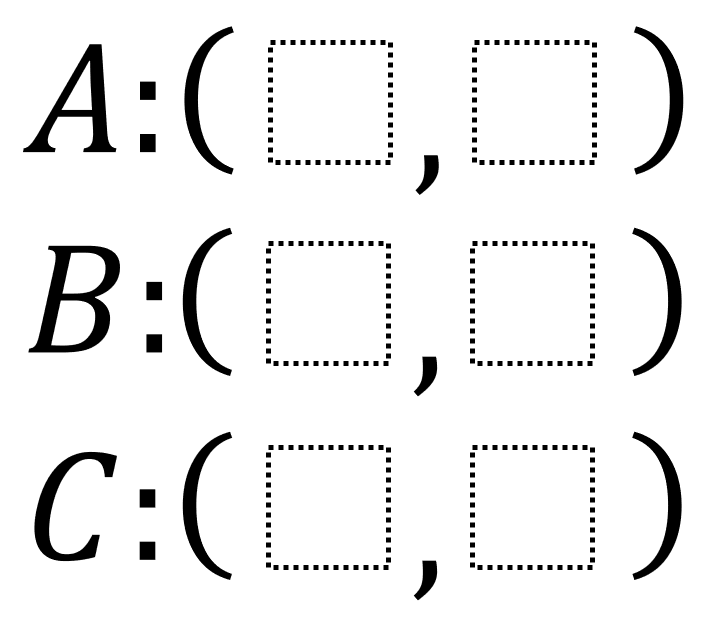# Area on a Coordinate Plane 1

Directions: Using the integers -9 to 9 at most one time each, fill in the boxes to create coordinates that represent the vertices of two triangles: one with an area of less than 55 units2 and one with an area of more than 55 units2. You may reuse all the integers each time.### Hint

How can you graphically estimate your triangle’s area before you calculate it?

There are many possible answers. One is:
Greater than 55 units2 which has an area 121.5 units2
(-9, -8), (0, 8), and (9, -3)

Less than 55 units2
(-3, 1), (0, 4), and (3, -4) which has an area 16.5 units2

Source: Robert Kaplinsky

## Similar Triangles 2

Directions: Using the digits 0 to 9 at most one time each, create two similar …